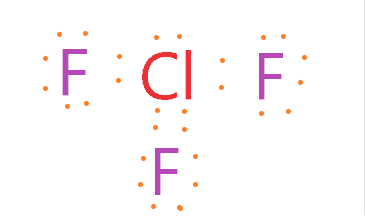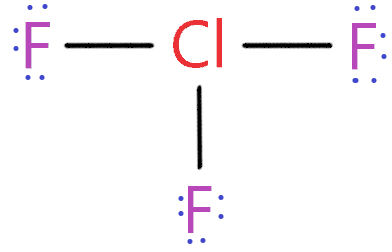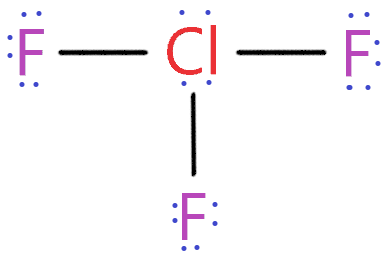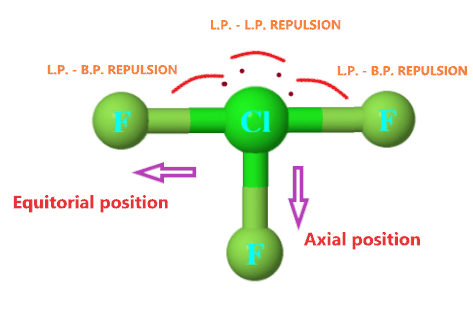# Chlorine trifluoride (ClF3) lewis dot structure, molecular geometry, polar or non-polar, bond angle

Home  > Chemistry Article > ClF3 lewis structure and its molecular geometryIn this article, we will discuss ClF3 lewis structure, molecular geometry, is it polar or non-polar, bond angle, hybridization, etc.

It is used in the semiconductor industry, rocket propellant, and military applications.

Chlorine trifluoride properties

• It is corrosive to metal and tissue.
• It boils at 53°F.
• It is a very strong oxidizing and fluorinating agent.
• ClF3 melting point is −76.34 °C and the boiling point is 11.75 °C.
• It has a molar mass of 92.45 g·mol−1
 Name of Molecule Chlorine trifluoride Chemical formula ClF3 Molecular geometry of ClF3 T-shaped Electron geometry of ClF3 Trigonal bipyramidal Hybridization Sp³d Lone pair 2 Total Valence electron in ClF3 lewis structure 28 The formal charge of ClF3 0
Page Contents

## How to draw ClF3 lewis structure

ClF3 lewis structure contains 3 fluorine atoms at the surrounding position and 1 chlorine atom at the central position. The lewis structure of ClF3 violates the octet as the central atom of it has more than 8 electrons in the outer shell.

ClF3 lewis dot structure contains a total of 11 lone pairs(3 on each fluorine atom and 2 on the chlorine atom). There is three bonded pair as well.

## Follow these steps to draw the stable ClF3 Lewis dot structure.

1. Count total valence electron in ClF3

In the first step, count all valence electrons present in this molecule. Both chlorine and fluorine belong to the 17th group in the periodic table.

⇒ Chlorine valence electron = 7

⇒ Fluorine valence electrons = 7*3      [∴F3]

Total valence electrons available for drawing the ClF3 lewis structure = 7 + 7*3 = 28 valence electrons.2. Find the least electronegative atom

Both chlorine and fluorine belong to the same group in the periodic table. And we need to find which atom has less electronegativity.

As electronegative decreases from up to down in the periodic table and chlorine situated below fluorine. So, we got our least electronegative atom.

Also, fluorine is the most electronegative element in chemistry, so, it always goes outside in the lewis diagram.

Place chlorine atom at the center in lewis diagram and fluorine atoms spaced evenly around it.3. Connect Chlorine and Fluorine with a single bond

Now after placing Chlorine at the center, we need to connect every outer atom to the central atom with the help of a single bond.As you see in this structure, we used three single bonds to connect the outer atom to the central atom.

So, we used 6 electrons from a total of 28 valence electrons.

∴ (28 – 6) = 22 valence electrons

Now we are left with 22 valence electrons more.

4. Locate the remaining valence electrons starting from the outer atom first

Now in this step, we need to place the remaining valence electrons starting from the outer atom first to complete the octet rule of every atom present in the ClF3 molecule.

So, we have 22 more valence electrons to work and Fluorine (outer atom) needs 8 electrons to complete its octet rule.

As each fluorine already shares 2 electrons with the help of a single bond. So, we need only 6 more electrons to complete each fluorine octet.As you see in this above structure, we placed 6 electrons around each fluorine.

⇒ (6 electrons × 3 fluorine atom) = 18 valence electron

We had 22 remaining valence electrons

∴ (22 – 18) = 4 electrons

So, now we are left with 4 more valence electrons.

Let’s use these remaining electrons around the central atom in the next step.

5. Complete central atom octet and make covalent bond if necessary

This is the final step for making the ClF3 Lewis structure.

We have 4 valence electrons that need to place around the chlorine central atom.### ClF3 lewis structure

By looking at the above structure, we see chlorine central atom has a total of 10 electrons around but for achieving octet, only 8 electrons are needed. Then why chlorine atom takes extra electrons?

This is because the lewis diagram is the representation of all the valence electrons around atoms within a molecule, so, we have 4 remaining valence electrons, but for completing the octet of chlorine atom only 2 electrons are needed as it already sharing 6 electrons through three single bonds.

Also, the Chlorine atom can expand the octet because it has d-orbitals in the third principal energy level, hence, it has an extra orbital(d-orbital) for additional electrons needed for bonding.

So, for fulfilling the requirement of the lewis diagram rule, we have to use all valence electrons within a molecule, it doesn’t matter if some atoms share more than 8 electrons because there can be exceptions in the octet rule.

We used all the valence electrons that are available for drawing the Lewis structure of ClF3.

So, we got the correct and best Chlorine trifluoride lewis structure.

Still, you can verify its stability by using the formal charge concept.

⇒ Formal charge of Cl = Valence electrons (7) – 1/2*Bonding electrons (6) – Lone pair of electrons (2*2)
= 7 – 3 – 4 = 0.

⇒ Formal charge of each F atom = Valence electrons (7) – 1/2*Bonding electrons (2) – Lone pair of electrons ( 2*3)
= 7 – 1 – 6 = 0.

The formal charge is zero on each atom(chlorine and fluorine) in the ClF3 Lewis structure. As “lower the formal charge, higher is the stability of lewis diagram”.

## What are the electron and molecular geometry of ClF3?

According to VSEPR (Valence shell electron pair repulsion theory), ClF3 molecular geometry is T-shaped and its electron geometry is trigonal bipyramidal.

“There are two lone pairs present on the central atom of ClF3 molecule Which is adjusted on the axial plane to maximize the bond angle and minimize the bond repulsion. Two F are adjusted on an equatorial position separated by an angle of 180°. And one is adjusted on the axial plane in a see-saw way. It acquires a trigonal bipyramid structure but lone pairs are not a part of the structure. Hence the structure of ClF3 is a T-shaped structure”.[Source]As you see in the above structure, two lone pairs on central atom repelling each other, also, they tried to repel bonded pair electrons as well, as a result, both fluorine atoms in the equatorial position pushed far apart from each other giving the look of T-Shaped structure.

Theoretically, We need ClF3 lewis’s structure to help to determine its shape. Because the lewis diagram helps us to identify how many lone pairs and bond pairs a molecule contains.

Let’s see step by step how to determine the molecular and electron geometry of ClF3.

1. Find the Number of lone pairs present on the central atom of the ClF3 lewis structure

First of all, we need to find how many lone pairs ClF3 central atom contains. As we see in the ClF3 lewis structure, Chlorine which is the central atom contains 2 lone pairs.

Or we can find lone pair in ClF3 by using the direct formula:

L.P = (V.E. – N.A.)/2

where L.P. = Lone pair on the central atom

⇒ V.E. = valence electron of that central atom

⇒ N.A. = Number of atoms attached to that central atom

∴ Here, Chlorine is the central atom that has 7 valence electrons and 3 fluorine atoms attached to it.

So, put these values in the formula to find lone pair.

∴ (7 – 3)/2

= 2 lone pairs on central atom(chlorine)

2. Find hybridization number of ClF3

Now we need to find the hybridization number of ClF3 so that we can determine its molecular and electron geometry.

Use the below Formula to find the Hybridization number of ClF3

H = N.A. + L.P.

where H = hybridization number

⇒ N.A. = Number of atoms attached to the central atom

⇒ L.P. = lone pairs on that central atom

Look at the ClF3 structure, three fluorine atoms attached to the central atom(Chlorine) and two lone pairs present on the central atom.

So, H = 3 + 2

= 5 is the hybridization number of ClF3

It means the hybridization of ClF3 is Sp³d.

3. Use VSEPR theory or AXN method to determine ClF3 molecular/electron geometry

This is the final step to determine the geometry of ClF3. So, According to the VSEPR chart if the molecule contains Sp³d hybridization and two lone pairs then the molecular geometry of that molecule is T-shaped and electron geometry is trigonal pyramidal.### ClF3 Molecular geometry or Shape

You can also use the AXN method to determine the molecular geometry or electron geometry of ClF3.

• A represents the central atom.
• X represents the bonded atoms to the central atom.
• N represents the lone pairs on the central atom

With the help of the ClF3 Lewis dot structure, we know chlorine is the central atom that contains 2 lone pairs and is attached to 3 bonded atoms.

So, the ClF3 formula becomes AX3N2.

According to the AX3N2 formula, ClF3 molecular geometry is T-shaped and electron geometry is trigonal pyramidal.

 Bonded atoms Lone pair Generic formula Hybridization Molecular geometry Electron geometry 1 0 AX S Linear Linear 2 0 AX2 Sp Linear Linear 1 1 AXN Sp Linear Linear 3 0 AX3 Sp² Trigonal planar Trigonal planar 2 1 AX2N Sp² Bent Trigonal planar 1 2 AXN2 Sp² Linear Trigonal planar 4 0 AX4 Sp³ Tetrahedral Tetrahedral 3 1 AX3N Sp³ Trigonal pyramid Tetrahedral 2 2 AX2N2 Sp³ Bent Tetrahedral 1 3 AXN3 Sp³ Linear Tetrahedral 3 2 AX3N2 Sp³d T-shaped Trigonal bipyramidal

VSEPR Chart

As ClF3 has 2 lone pair or 3 bond repulsion units and it formed  T-shaped or trigonal pyramidal geometry, Its F—Cl—F involving the axial atoms bond angle is 175º and F—Cl—F involving the one axial atom and one equatorial bond angle is around 90º.

## Chlorine trifluoride polarity: Is ClF3 polar or non-polar?

Is ClF3 polar or non-polar? Still don’t know? ClF3 is a polar molecule because it has an asymmetrical shape and the presence of 2 lone pair electrons leading to an unequal distribution of charge making this molecule polar in nature.

Let’s understand in depth why ClF3 is polar in nature with the help of three factors.

Three factors that indicate the polarity of ClF3

1. Electronegativity:

Electronegativity shows the tendency of an atom to pulling electrons to itself. The higher the difference of electronegativity between atoms greater is the polarity of that atom.

Clearly, in the ClF3 molecule, Fluorine is more electronegative than chlorine.

The electronegativity value of Chlorine is 3.16 and for fluorine, it is 4. The difference between the electronegativity of Fluorine and chlorine is more than 0.5.

Hence ClF3 is polar in nature.

2. Dipole moment

This is an accurate way to determine whether ClF3 is polar or non-polar. If the molecule has some net dipole moment then that molecule is polar in nature.

The higher the dipole moment of the molecule greater is the polarity strength of that molecule.

The dipole moment is induced by the formation of negative and positive charges in the molecule.Dipole moment diagram of ClF3

As you see in the above figure, dipole vector direction towards Fluorine. Because fluorine is more electronegative than Chlorine. Hence it attracts electrons towards itself and develops a partial negative charge.

The partial positive charge was also developed by Chlorine and we know separation of positive and negative charge lead to some dipole moment of the molecule.

So, these dipoles can’t be canceled out. Thus it making ClF3 a polar molecule in nature.

3. Geometrical or molecular shape:

The geometry of ClF3 has a great influence on its polarity. As we know asymmetrical shape causes to become a molecule polar in nature.

From the lewis structure of ClF3, we know 2 lone pair present on its central atom thus it making the shape of ClF3 bent and causing unequal distribution of charges which induces a permanent dipole between atoms.

Also, the electron geometry of ClF3 is trigonal bipyramidal, and the molecular shape is T-Shaped which is the non-symmetrical shape.

Hence all these factors help to know whether ClF3 is polar or non-polar.

## FAQ

### How many lone pairs and bond pairs does Chlorine contain according to the ClF3 lewis structure?

The number of lone pairs on the central atom(chlorine) is two, and the bond pair which is attached to the central atom is three.

### Why Chlorine shared 10 electrons to complete its octet in the ClF3 Lewis dot structure?

As we know chlorine only needs 8 electrons to complete its octet rule despite this chlorine shares 10 electrons with other atoms and violates the octet rule.

Because Chlorine is an expanded octet in the Lewis structure of ClF3. And fluorine will not take more than 8 electrons as it is already fully stable leaving chlorine with 4 extra electrons.

Hence these extra 4 electrons act as lone pair electrons on Chlorine central atom.

### Why molecule geometry of ClF3 is T-shaped?

Because two lone pairs which are present on the Chlorine in the ClF3 lewis structure acquire the equatorial position as they demand more space than bond pairs.

Hence it makes the molecular shape of ClF3 look-like T-shaped.

## Summary

• Hybridization of ClF3 is Sp³d.
• The molecular geometry of ClF3 is T-shaped and electron geometry is Trigonal bipyramidal.
• The total valence electron present available for drawing the ClF3 Lewis structure is 28
• According to the ClF3 Lewis structure, 2 lone pairs and 3 bonded pairs are present in this molecule.
• ClF3 is polar in nature.
##### Subscribe to Blog via Email

Join 2 other subscribers

Share it...